### 最终效果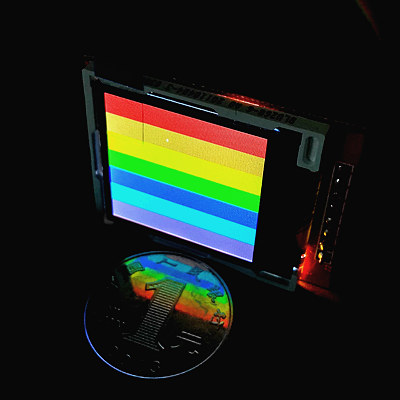### 硬件

• 1.44英寸 spi接口 128x128液晶屏幕 X 1（淘宝10-20元）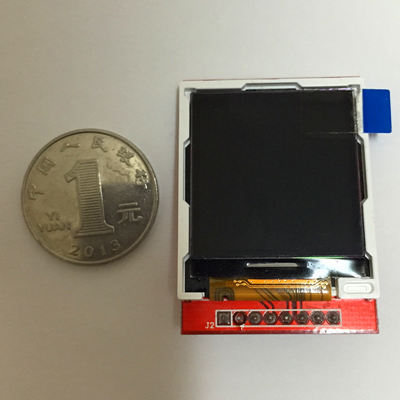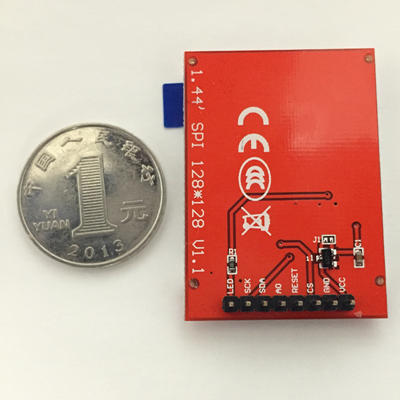### 先上代码看效果

#!/usr/bin/env python

import RPi.GPIO as GPIO
import time

cs=23        # 片选
rs=17        # 数据 / 命令 切换
sda=13        # 数据
scl=19        # 时钟
reset=27    # 复位

# 传输byte
def setByteData(data):
# print ""
# print "S-----------setByte---------------:", hex(data)
for bit in range(0,8):
# 传入的数字从高位到低位依次判断是否为1，若为1则设置高电平，否则设置低电平
# 判断的方法是先向左移位，把要判断的位移动到最高位然后跟0x80（1000 0000）相与，
# 如果结果仍然是0x80（1000 0000）就表示最高位是1，否则最高位就是0
if ((data<<bit) & 0x80 == 0x80):
setBitData(True)
# print "1",
else:
setBitData(False)
# print "0",
# print ""
# print "E-----------setByte---------------"

def setBitData(data):
GPIO.output(scl, False)
GPIO.output(sda, data)
GPIO.output(scl, True)

def write_command(cmd):
GPIO.output(cs, False)
GPIO.output(rs, False)
setByteData(cmd)
GPIO.output(cs, True)

def write_data(data):
GPIO.output(cs, False)
GPIO.output(rs, True)
setByteData(data)
GPIO.output(cs, True)

def write_data_16bit(dataH, dataL):
write_data(dataH)
write_data(dataL)

def lcd_reset():
GPIO.output(reset, False)
time.sleep(0.1)
GPIO.output(reset, True)
time.sleep(0.1)

def lcd_init():
lcd_reset()

write_command(0x11) # Exit Sleep
time.sleep(0.02)
write_command(0x26) # Set Default Gamma
write_data(0x04)
write_command(0xB1)# Set Frame Rate
write_data(0x0e)
write_data(0x10)
write_command(0xC0) # Set VRH1[4:0] & VC[2:0] for VCI1 & GVDD
write_data(0x08)
write_data(0x00)
write_command(0xC1) # Set BT[2:0] for AVDD & VCL & VGH & VGL
write_data(0x05)
write_command(0xC5) # Set VMH[6:0] & VML[6:0] for VOMH & VCOML
write_data(0x38)
write_data(0x40)

write_command(0x3a) # Set Color Format
write_data(0x05)
write_command(0x36) # RGB
write_data(0xc8)

write_data(0x00)
write_data(0x00)
write_data(0x00)
write_data(0x7F)
write_data(0x00)
write_data(0x00)
write_data(0x00)
write_data(0x7F)

write_command(0xB4)
write_data(0x00)

write_command(0xf2) # Enable Gamma bit
write_data(0x01)
write_command(0xE0)
write_data(0x3f)# p1
write_data(0x22)# p2
write_data(0x20)# p3
write_data(0x30)# p4
write_data(0x29)# p5
write_data(0x0c)# p6
write_data(0x4e)# p7
write_data(0xb7)# p8
write_data(0x3c)# p9
write_data(0x19)# p10
write_data(0x22)# p11
write_data(0x1e)# p12
write_data(0x02)# p13
write_data(0x01)# p14
write_data(0x00)# p15
write_command(0xE1)
write_data(0x00)# p1
write_data(0x1b)# p2
write_data(0x1f)# p3
write_data(0x0f)# p4
write_data(0x16)# p5
write_data(0x13)# p6
write_data(0x31)# p7
write_data(0x84)# p8
write_data(0x43)# p9
write_data(0x06)# p10
write_data(0x1d)# p11
write_data(0x21)# p12
write_data(0x3d)# p13
write_data(0x3e)# p14
write_data(0x3f)# p15

write_command(0x29) #  Display On
write_command(0x2C)

def show_single_color(DH,DL):
for i in xrange(0,128):
for j in xrange(0,128):
write_data_16bit(DH,DL)

try:
GPIO.setmode(GPIO.BCM)
GPIO.setup(cs, GPIO.OUT)
GPIO.setup(rs, GPIO.OUT)
GPIO.setup(sda, GPIO.OUT)
GPIO.setup(scl, GPIO.OUT)
GPIO.setup(reset, GPIO.OUT)

lcd_init()
write_command(0x2C)

show_single_color(0xf8,0x00) # 红色背景

while True:
pass

except KeyboardInterrupt:
pass

# 清理GPIO口
GPIO.cleanup()

LCD3.3V(不可以是5V！！)
SCKGPIO19
SDAGPIO13
AOGPIO17
RESETGPIO27
CSGPIO23
GNDGND
VCC3.3V(不可以是5V！！)

sudo python prog.py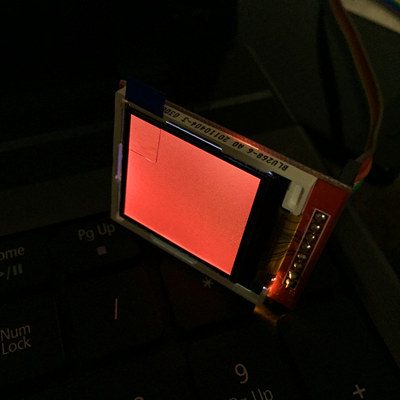### 原理说明

def write_command(cmd):
GPIO.output(cs, False)
GPIO.output(rs, False)
setByteData(cmd)
GPIO.output(cs, True)

def write_data(data):
GPIO.output(cs, False)
GPIO.output(rs, True)
setByteData(data)
GPIO.output(cs, True)

• 0x2A：指定绘图指针的列起始和结束地址（X坐标范围）
指令内容是4个字节的32位数据，从高位到低位分别是

• 0x2B：指定绘图指针的行起始和结束地址（Y坐标范围）
指令内容是4个字节的32位数据，从高位到低位分别是

write_command(0x2A) # Set Column Address
write_data(0x00)
write_data(0x00)
write_data(0x00)
write_data(0x7F)
write_data(0x00)
write_data(0x00)
write_data(0x00)
write_data(0x7F)
• 0x2C：在当前指针位置（X,Y坐标）绘制指定颜色的像素点
指令内容是2个字节的颜色数据。

write_command(0x2C)
show_single_color(0xf8,0x00) # 红色背景
...
...
def show_single_color(DH,DL):
for i in xrange(0,128):
for j in xrange(0,128):
write_data_16bit(DH,DL)

### 关于颜色的补充说明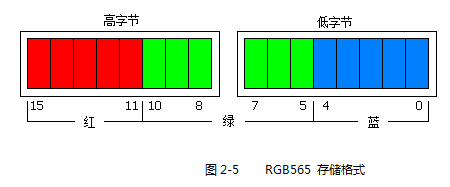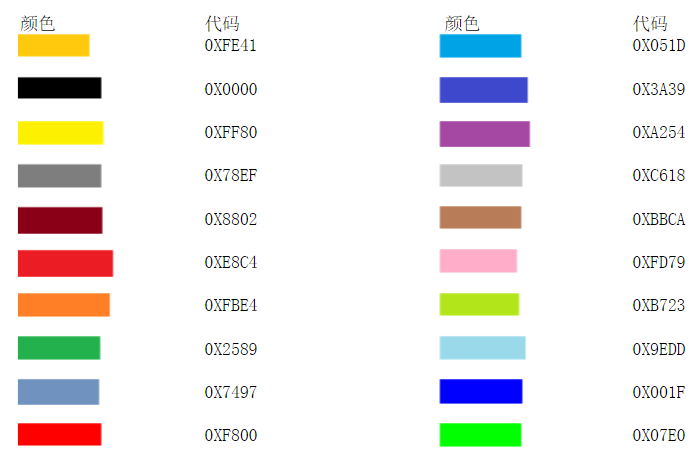• 4k Colors, RGB 4,4,4
3AH=“03h”
write_command(0x3a)
write_data(0x03)
• 65k Colors, RGB 5,6,5
3AH=“05h”（本文使用的格式）
write_command(0x3a)
write_data(0x05)
• 262k Colors, RGB 6,6,6
3AH=“06h”
write_command(0x3a)
write_data(0x06)

### 资源下载

Last modification：April 30th, 2021 at 05:12 am